×#### Thank you for registering.

One of our academic counsellors will contact you within 1 working day.

Click to Chat

1800-1023-196

+91-120-4616500

CART 0

• 0

MY CART (5)

Use Coupon: CART20 and get 20% off on all online Study Material

ITEM
DETAILS
MRP
DISCOUNT
FINAL PRICE
Total Price: Rs.

There are no items in this cart.
Continue Shopping• Complete JEE Main/Advanced Course and Test Series
• OFFERED PRICE: Rs. 15,900
• View Details

```Chapter 2: Exponents Of Real Numbers Exercise – 2.1

Question: 1

Simplify the following:

(i) 3(a4 b3)10 × 5 (a2 b2)3

(ii) (2x-2 y3)3Solution:

(i) 3(a4 b3)10 × 5 (a2 b2)3

= 3(a40 b30) × 5(a6 b6)

= 15 (a46 b36)

(ii) (2x-2 y3)3

(23 × -2 × 3 y3 × 3) = 8x-6y9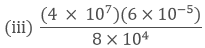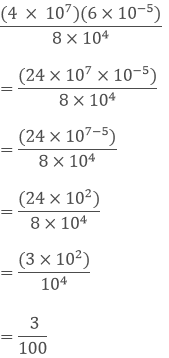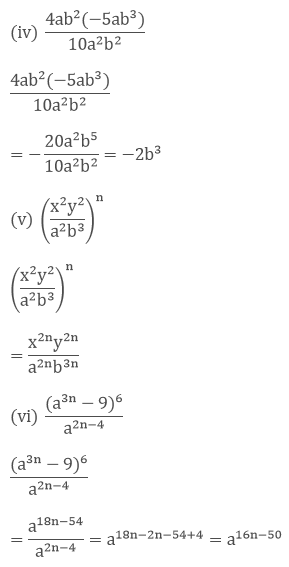Question: 2

If a = 3 and b = - 2, find the values of:

(i) aa + bb

(ii) ab + ba

(iii) ab + ba

Solution:

(i) We have,

aa + bb

= 33 + (−2) −2

= 33 + (−1/2)2

= 27 +1/4

= 109/4

(ii) ab + ba

= 3−2 + (−2)3

= (1/3)2 + (−2)3

= 1/9 – 8

= −(71/9)

(iii) We have,

ab + ba

= (3 + (−2))3(−2)

= (3 − 2))−6

= 1−6 = 1

Question: 3

Prove that: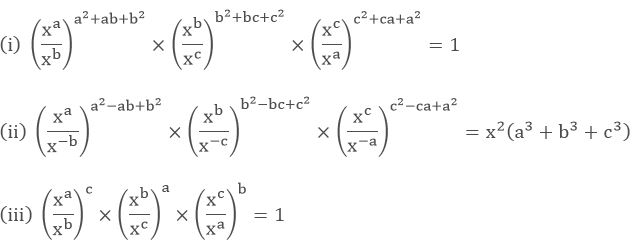Solution:

(i) To prove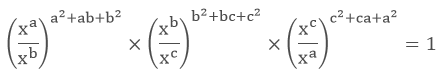Left hand side (LHS) = Right hand side (RHS) Considering LHS,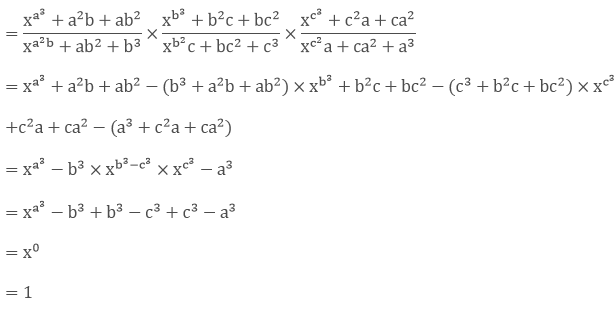Or, Therefore, LHS = RHS Hence proved

(ii) To prove,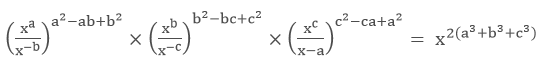Left hand side (LHS) = Right hand side (RHS) Considering LHS,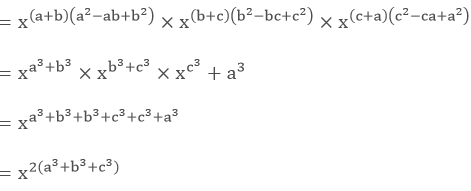Therefore, LHS = RHS Hence proved

(iii) To prove,Left hand side (LHS) = Right hand side (RHS) Considering LHS,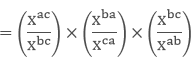= xac−bc × xba−ca × xbc−ab

= xac − bc + ba − ca + bc − ab

= x0

= 1

Therefore, LHS = RHS

Hence proved

Question: 4

Prove that: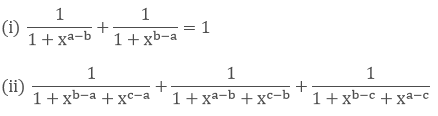Solution:Left hand side (LHS) = Right hand side (RHS) Considering LHS,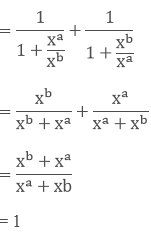Therefore, LHS = RHS Hence provedLeft hand side (LHS) = Right hand side (RHS) Considering LHS,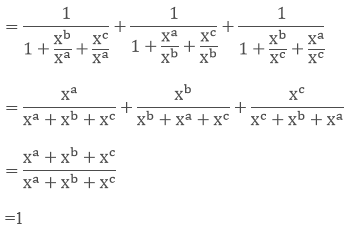Therefore, LHS = RHS Hence proved

Question: 5

Prove that:Solution:

(i) To prove,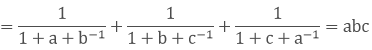Left hand side (LHS) = Right hand side (RHS) Considering LHS,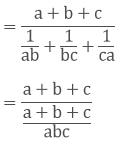= abc

Therefore, LHS = RHS Hence proved

(ii) To prove,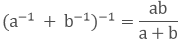Left hand side (LHS) = Right hand side (RHS) Considering LHS,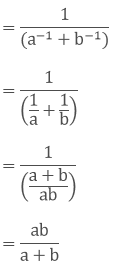Therefore, LHS = RHS

Hence proved

Question: 6

If abc = 1, show that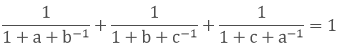Solution:

To prove,Left hand side (LHS) = Right hand side (RHS) Considering LHS,We know abc = 1

c = 1/ab

By substituting the value c in equation (1), we getTherefore, LHS = RHS Hence proved

Question: 7

Simplify: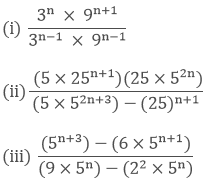Solution: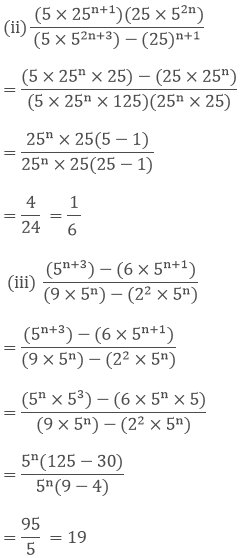Question: 8

Solve the following equations for x:

(i) 72x+3 = 1

(ii) 2x+1 = 4x−3

(iii) 25x+3 = 8x+3

(iv) 42x = 1/32

(v) 4x−1 × (0.5)3−2x = (1/8)x

(vi) 23x−7 = 256

Solution:

(i) We have,

⟹ 72x+3 = 1

⟹ 72x+3 = 70

⟹ 2x + 3 = 0

⟹ 2x = -3

⟹ x = −3/2

(ii) We have,

= 2x+1 = 4x−3

= 2x+1 = 22x−6

= x + 1 = 2x - 6

= x = 7

(iii) We have,

= 25x+3 = 8x+3

= 25x+3 = 23x+9

= 5x + 3 = 3x + 9

= 2x = 6

= x = 3

(iv) We have,

= 42x = 1/32

= 24x = 1/25

= 24x = 2−5

= 4x = - 5

x = -5/4

(v) We have,

4x−1 × (0.5)3−2x = (1/8)x

22x−2 × (1/2)3−2x = (1/2)3x

22x−2 × 22x−3 = (1/2)3x

22x−2+ 2x−3 = (1/2)3x

24x−5 = 2−3x

4x-5 = -3x

7x = 5

x = 5/7

(vi) 23x−7 = 256

23x−7 = 28

3x - 7 = 8

3x = 15

x = 5

Question: 9

Solve the following equations for x:

(i) 22x − 2x+3 + 24 = 0

(ii) 32x+4 + 1 = 2 × 3x+2

Solution:

(i) We have, ⟹ 22x − 2x+3 + 24 = 0

⟹ 22x + 24 = 2x.23

⟹ Let 2x = y

⟹ y2 + 24 = y × 23

⟹ y2 − 8y + 16 = 0

⟹ y2 − 4y − 4y + 16 = 0

⟹ y(y - 4) - 4(y - 4) = 0

⟹ y = 4

⟹ x2 = 22

⟹ x = 2

(ii) We have,

32x+4 + 1 = 2 × 3x+2

(3x+2)2 + 1 = 2 × 3x+2

Let 3x+2 = y

y2 + 1 = 2y

y2 − 2y + 1 = 0

y2 − y − y + 1 = 0

y(y − 1) − 1(y − 1) = 0

(y − 1)(y − 1) = 0

y = 1

Question: 10

If 49392 = a4b2c3, find the values of a, b and c, where a, b and c, where a, b, and c are different positive primes.

Solution:

Taking out the LCM, the factors are 24, 32 and 73 a4b2c3 = 24, 32 and 73

a = 2, b = 3 and c = 7 [Since, a, b and c are primes]

Question: 11

If 1176 = 2a × 3b × 7c, Find a, b, and c.

Solution:

Given that 2, 3 and 7 are factors of 1176.

Taking out the LCM of 1176, we get 23 × 31 × 72 = 2a × 3b × 7c

By comparing, we get

a = 3, b = 1 and c = 2.

Question: 12

Given 4725 = 3a × 5b × 7c, find

(i) The integral values of a, b and c

(ii) The value of 2−a × 3b × 7c

Solution:

(i) Taking out the LCM of 4725, we get

33 × 52 × 71 = 3a × 5b × 7c

By comparing, we get

a = 3, b = 2 and c = 1.

(ii) The value of 2−a × 3b × 7c

Sol:

2-a × 3b × 7c = 2−3 × 32 × 71

2−3 × 32 × 71 = 1/8 × 9 × 7

63/8

Question: 13

If a = xyp−1, b = xyq−1 and c = xyr−1, prove that aq−r br−p cp−q = 1

Solution:

Given, a = xyp−1, b = xyq−1 and c = xyr−1

To prove, aq−rbr−pcp−q = 1

Left hand side (LHS) = Right hand side (RHS)

Considering LHS, = aq−r br−p cp−q ...... (i)

By substituting the value of a, b and c in equation (i), we get

= (xyp−1)q−r(xyq−1)r−p(xyr−1)p−q

= xypq−pr−q+rxyqr−pq−r+pxyrp−rq−p+q

= xypq−pr−q+ r+qr−pq−r+p+rp−rq−p+q

= xy0

= 1
```### Course Features

• 728 Video Lectures
• Revision Notes
• Previous Year Papers
• Mind Map
• Study Planner
• NCERT Solutions
• Discussion Forum
• Test paper with Video Solution